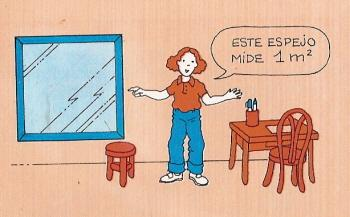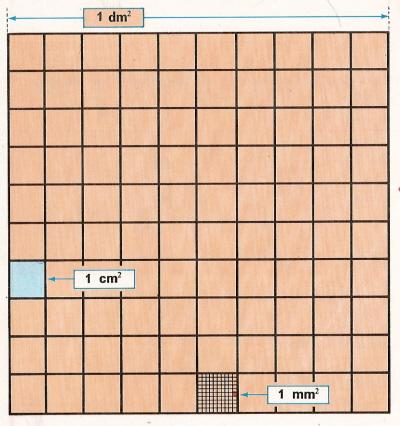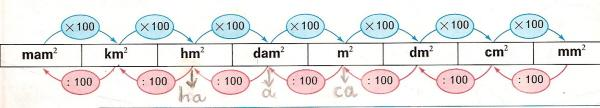Unit of surface1. The square meter.

The square meter is the area of a square whose sides measure exactly one meter. Its symbol is: m.2.

2. Multiples of the square meter.

These are:

1 square decameter is equal to 100 square meters: 1 dam2 = 100 m2 .
1 square hectometer is equal to 100 00 square meters: 1 hm2 = 100 00 m2.
1 square kilometer is equal to 100 00 00 square meters: 1 km2 = 100 00 00 m2.
1 square myriameter is equal to 100 00 00 00 square meters: 1 mam2 = 100 00 00 00 m2.
To measure fields, we use agricultural units. These are:
1 hectare is equal to hm2: ha = hm2 = 100 00 m2.
1 area is equal to dam2: a = dam2 = 100 m2.
1 centiare is equal to m2: ca = m2 = 1 m2.

The units of surface increase and decrease from 100 to 100.
The superior unit is 100 more than the lower.

It answers these questions in square meters:

 2 dam2 = 7 km2 = 6 hm2 =3. - Submultiples of the square meter

These are:

1 square decimeter is equal to 0.01 square meters: 1 dm2 = 0,01 m2. 1 m2 has 100 dm2.
1 square centimeter is equal to 0.0001 square meters: 1 cm2 = 0,00 01 m2. El m2 has 100 00 cm2.
1 square millimeter is equal to 0.000001 square meters: 1 mm2 = 0,00 00 01 m2. El m2 has 100 00 00 m2.

The units of surface increase and decrease from 100 to 100.
The lower unit is 100 less than the superior.

It answers these questions in square meters:

 6 cm2 = 2 mm2 = 5 dm2 =4. Conversion of unit

Each unit of surface is 100 times greater than the immediate lower and 100 times less than the immediate superior.

To convert from dam2 to m2 we multiply by 100 or we move the decimal point two places to the right.
Examples: 7 dam2 = 700 m2; 73,25 7 hm2 = 73 25,7 dam2 = 73 25 70 m2.

To convert from m2 to dam2, we divide by 100 or we move the decimal point two places to the left.
Examples: 3 m2 = 0,03 dam2; 14 68 m2 = 14,68 dam2 = 0,14 68 hm2 = 0,00 14 68 km2.

It answers these questions in square meters::

 8 dam2 = 6,3 km2 = 235 cm2 =

5. Converting from complex to non-complex

To convert from surface complex to a non-complex of lower order, we write the numbers of successive orders, reserving two places for each order and placing zeros in the empty places.

Example: 5 hm2, 18 dam2 and 25 m2 can be written as: 5 18 25 m2

It answers these questions in square meters:

 3 ha, 7 a y 2 ca = 8 km2, 17 dam2, y 3 dm2 = 41 hm2, 25 m2 y 7 cm2 =

6. Converting from non-complex ( 1 25 m2 ) to complex ( 1 dam2 and 25 m2 ).

To convert from surface non-complex to complex, we separate the numbers in pairs, from the decimal point in both places (before and after the decimal point), putting the corresponding names to their orders.

For example: if the area of a field is 60 26,21 8 m2, we complete the orders 60 26,21 80 m2, and then it can be written as: 60 dam2, 26 m2, 21 dm2 and 80 cm2.

Examples:
30 00,02 m2 = 30 dam2 and 2 dm2
73 00 00,00 00 31 m2 = 73 hm2 and 31 mm2.
5,7 mam2 = 5 mam2 and 70 km2.

7. Problems.

Do these problems on a paper and write the solution:

 1. If the m2 of a land cost 2 euros, how many money will I pay if I buy a land of 7 ha? 2. One province is 14725 km2. How many areas are there? 3. A field of 12350 m2 is divided into four equal parts. How many dam2 does each part measure? 4. The floor of a room is 15.598 m2 and has 55 floor tiles. How many cm2 does each floor tile measure? 5. A courtyard has 25 rows of tiles with 37 tiles each one of them. The courtyard is 1 dam2, 66 m2 and 50 dm2. How many dm2 does each tile measure? 6. How many standing people can be on a courtyard of 3 dam2 and 60 m2 if each person occupies an area of 20 dm2? 7. We have a farm and we have sold 2/5 to 0.33 euro per m2 and the rest of the farm have sold to 30.1 euros per dam2. How many money did we obtain from the sale? 8. On day of rain, 82 liters of water have fallen in a square meter. How many hectoliters of water have fallen in a field of 25 ha and 87 a? 9. The surface of the Earth is 5101000 mym2 and 3/4 is occupied by the oceans. How many km2 do the continents take up? 10. The largest island on earth is the Greenland and it measures 2180000 km2 and one of the smallest island is the Cabrera that has 2000 ha. How many times does the Cabrera fit in Greenland?

 | Educational applications | Mathematics | In Spanish  | To print

®Arturo Ramo García.-Record of intellectual property of Teruel (Spain) No 141, of 29-IX-1999
Plaza Playa de Aro, 3, 1ş DO 44002-TERUEL